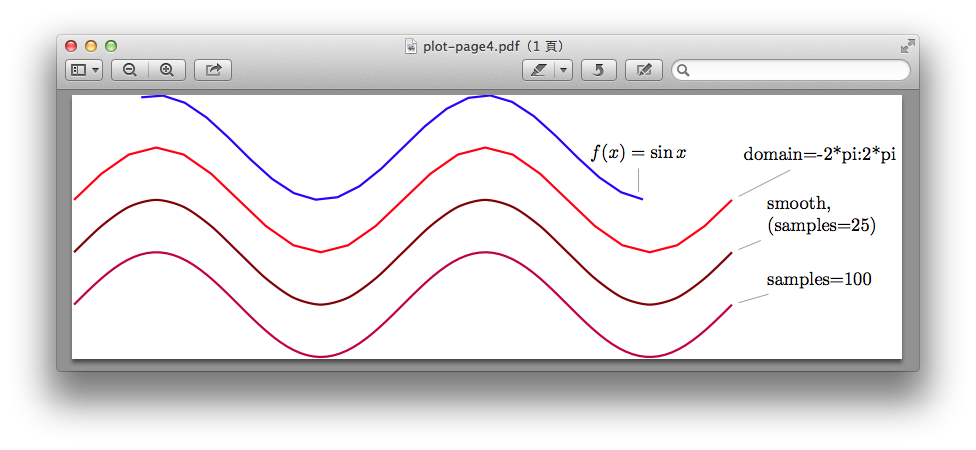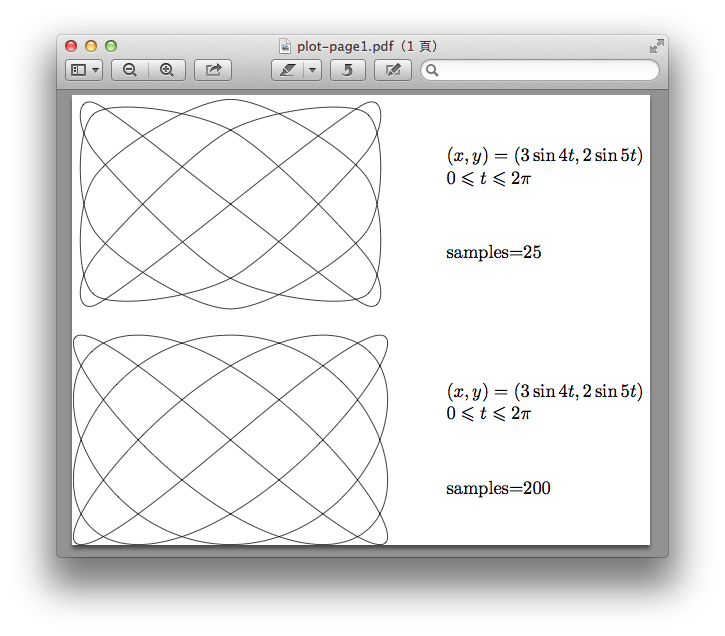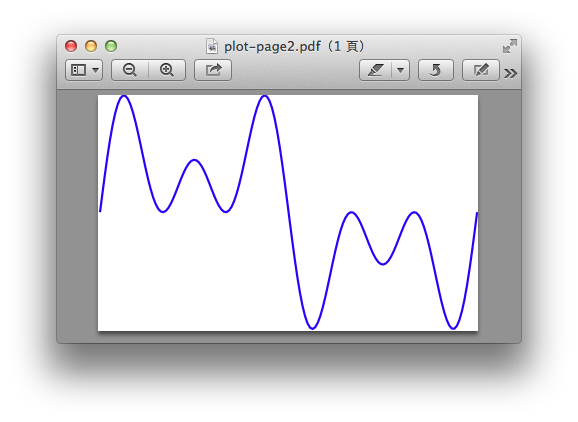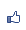Contents ...
udn網路城邦
TiKz plot指令畫數學函數二 使用pgfmath套件
2015/04/23 16:04

TiKz套件會自動載入pgfmath package，這裡面提供了很多函數工具。如果在latex文件中需要使用這些函數工具做運算時，則是要載入這個package：

\usepackage{pgfmath}

\pgfmathparse{2^5}\pgfmathresult

pgfmath 提供了哪些運算元和函數，可以看tikz文件的89節到93節的內容。或是直接看pgfmath 的套件說明。在tikz中，這些運算全部都在一組大括號中，如：

{2*sin(3 r)+cos(1.5 r)}

{e} 數學常數

{pi}圓周率

variable=marco, 預設值是\x。

domain=start:end ，預設值是－5:5。設定變數範圍：一個數學函數，是無窮個數值的集合，而一個圖案，有版面大小的限制。所以要畫數學函數圖形，是個數值範圍的限制。有這個，才能在一個範圍內畫出圖形。

samples=numer 取樣數，預設值25

samples at =sample list，可以指定用何值取樣，可以配合foreach指令，但這命令和domain 和 samples衝突。

\draw[help lines] (-5,-4) grid (5,2);

\draw plot(\x, {sin(\x r)}); 基本上就能畫出一個正弦函數圖, 下例藍。

\draw[red] plot[domain=-2*pi:2*pi](\x,{sin(\x r)});

\draw[red!50!black] plot [domain=-2*pi:2*pi, smooth](\x,{sin(\x r)});

\draw[draw=purple] plot [domain=-2*pi:2*pi, samples=100](\x,{sin(\x r)});\draw plot [domain=0:2*pi, smooth]({3*sin(4*\x r}, {2*sin(5*\x r)});declare function={ 函數命令;....;}

/draw [x=0.02cm,y=2cm,draw=blue, very thick] plot [domain=0:360, samples=150,

declare function={

sines(\t,\a,\b)=1+0.5*(sin(\t)+sin(\t*\a)+sin(\t*b));}](\x, {snes(\x,3,5)});declare function 也可以更複雜一些這是說明文件上面的例子，模擬聲音的函數：

\draw[help lines](0,0) grid (0,4);

\draw (0,2)-- [x=0.02cm,y=2cm, draw=red, very thick] plot [domain=0:360, samples=150, smooth, declare function={

excitation(\t,\w)=sin(\t*\w);

noice=rnd-0.5;

source(\t)=excitation(\t*20)+noise;

filter(\t)=2-abs(sin(mod(\t,90)));

speech(\t)=2+source(\t)*filter(\t);}] (\x,{speech(\x)});\draw plot [domain=0:720, samples=200,smooth, declare function ={

xpartt(\R,\r,\a)=(\R-\r)*sin(\a)+\r*sin((-\r/\R)*\a);

ypartt(\R,\r,\a)=(\R-\r)*cos(\a)+\r*cos((-\r/\R)*\a);}]

({xpartt(2/9, 7/9, \x)},{ypartt(2/9,7/9,\x)});